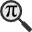## General

Display information for equation id:math.286743.31 on revision:286743

* Page found: Talk:Covariance matrix (eq math.286743.31)

(force rerendering)

Cannot find the equation data in the database. Fetching from revision text.

Occurrences on the following pages:

Hash: 262d5ec61d4727236470a56c2e8433ef

TeX (original user input):

\mu_i


TeX (checked):

\mu _{i}


### LaTeXML (experimental; uses MathML) rendering

MathML (822 B / 328 B) :${\displaystyle\mu_{i}}$
<math xmlns="http://www.w3.org/1998/Math/MathML" id="p1.1.m1.1" class="ltx_Math" alttext="{\displaystyle\mu_{i}}" display="inline">
<semantics id="p1.1.m1.1a">
<msub id="p1.1.m1.1.3" xref="p1.1.m1.1.3.cmml">
<mi id="p1.1.m1.1.1" xref="p1.1.m1.1.1.cmml">μ</mi>
<mi id="p1.1.m1.1.2.1" xref="p1.1.m1.1.2.1.cmml">i</mi>
</msub>
<annotation-xml encoding="MathML-Content" id="p1.1.m1.1b">
<apply id="p1.1.m1.1.3.cmml" xref="p1.1.m1.1.3">
<csymbol cd="ambiguous" id="p1.1.m1.1.3.1.cmml">subscript</csymbol>
<ci id="p1.1.m1.1.1.cmml" xref="p1.1.m1.1.1">μ</ci>
<ci id="p1.1.m1.1.2.1.cmml" xref="p1.1.m1.1.2.1">i</ci>
</apply>
</annotation-xml>
<annotation encoding="application/x-tex" id="p1.1.m1.1c">{\displaystyle\mu_{i}}</annotation>
</semantics>
[/itex]


SVG image empty. Force Re-Rendering

SVG (2.024 KB / 1.052 KB) :

### MathML with SVG or PNG fallback (recommended for modern browsers and accessibility tools) rendering

SVG image empty. Force Re-Rendering

SVG (0 B / 8 B) :

PNG (0 B / 8 B) :

$\mu _{i}$## Translations to Computer Algebra Systems

### Translation to Maple

In Maple: mu[i]

i: You use a typical letter for a constant [the imaginary unit == the principal square root of -1].

We keep it like it is! But you should know that Maple uses I for this constant.

If you want to translate it as a constant, use the corresponding DLMF macro \iunit

### Translation to Mathematica

In Mathematica: Subscript[\[Mu], i]

i: You use a typical letter for a constant [the imaginary unit == the principal square root of -1].

We keep it like it is! But you should know that Mathematica uses I for this constant.

If you want to translate it as a constant, use the corresponding DLMF macro \iunit

## Similar pages

Calculated based on the variables occurring on the entire Talk:Covariance matrix page

## Identifiers

• $\mu _{i}$### MathML observations

0results

0results

no statistics present please run the maintenance script ExtractFeatures.php

0 results

0 results